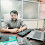# Pickling and Unpickling tutorials in Python?

2

Pickling:-

It means to convert object type data to stream, which means if we want to write object content into the file then we use this concept.

Python provides a pickle module to implement picking features.

import pickle

has a dump() and dumps() method to pickle data.

pickling means Object serialization and unpickling means object deserialization.

pickling and unpickling is used to transfer the data from one machine to another machine.

Example of Pickling?

import pickle

lst = ["C","C++","Java",".NET","PHP"]

f = open("d://coursedetails.txt","wb")

pickle.dump(lst,f)

f.close()

Example of Unpickling?

import pickle

f = open("d://coursedetails.txt","rb")

print(s)

f.close()

Example of dumps() and loads() in Python:-
Note:-  if we want to pickle real-time objects then we use dumps() and unpickle to real-time data we use loads().
import pickle
class Student:
rno=1001
sname="xyz"
x = ["c","CPP","JAVA"]
stu = Student()
st=pickle.dumps(stu)    #object to stream
print("Pickled Object is ",st)
s = pickle.loads(st)   # stream to object
print(s.x)
print(s.rno)
print(s.sname)
Tags

1.# Wap to find area of circle , triangle, ractangle using pickling & unpickling

import pickle
class Circle:
def accept(self,a):
self.a=a
def dis(self):
print("Area Of Circle : %d"%(3.14*self.a*self.a))
class Triangle(Circle):
def accept1(self,b):
self.b=b
def dis1(self):
print("Area Of Triangle : ",((self.a*self.b)/2))
class Ract(Triangle):
def accept2(self):
self.area=self.a*self.b
def dis2(self):
print("Area Of Ractangle : ",self.area)
f=open ('demo2.txt','wb')

a1=Circle()
a1.accept(a=5)
a1=Triangle()
a1.accept(a=5)
a1.accept1(b=10)
a1=Ract()
a1.accept(a=12)
a1.accept1(b=8)
a1.accept2()
pickle.dump(a1,f)
print("Pickling Done!!")
f=open ('demo2.txt','rb')

print("Unpickling Done!!")
obj.dis()
obj.dis1()
obj.dis2()

2.import pickle
class Student:
rno=1001
subject=['phy','chem','maths','english','hindi']

obj = Student()
data=pickle.dumps(obj)
f = open("picklemultiple.txt","wb")
f.write(data)
f.close()

f = open("picklemultiple.txt","rb")